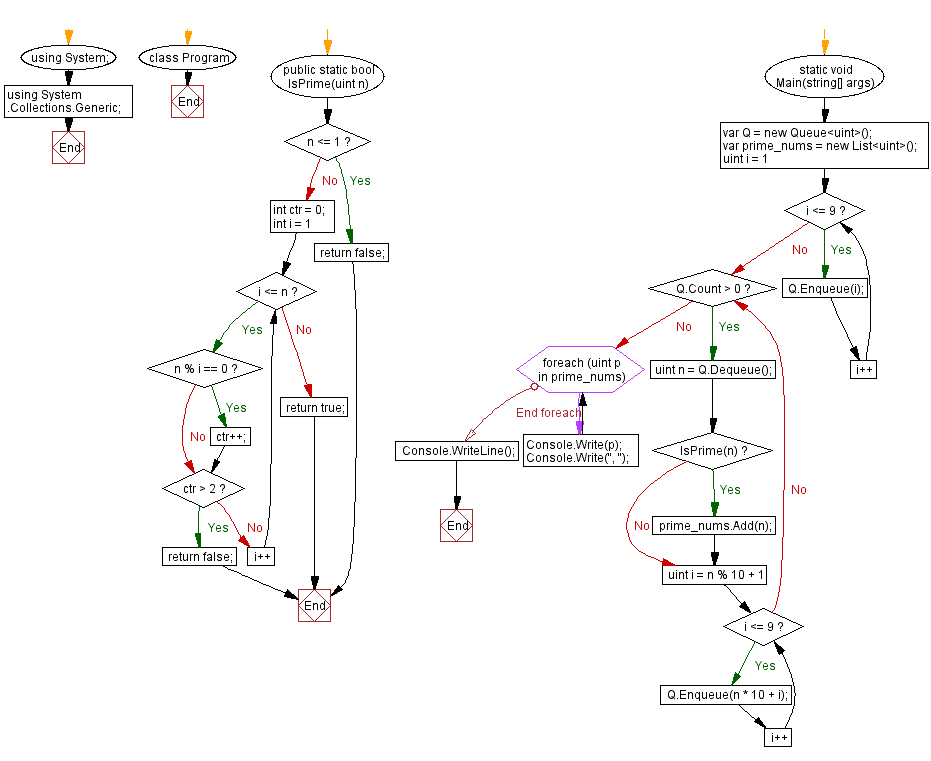﻿ C# - Prime number in strictly ascending decimal digit order

# C#: Prime number in strictly ascending decimal digit order

## C# Sharp Basic: Exercise-99 with Solution

Write a C# Sharp program to create and display all prime numbers in strict ascending decimal digit order.

Sample Data:
2, 3, 5, 7, 13, 17, 19, 23, 29, 37, 47, 59, 67, 79,

Sample Solution:

C# Sharp Code:

``````using System;
using System.Collections.Generic;

namespace exercises
{
class Program
{
// Method to check if a number is prime
public static bool IsPrime(uint n)
{
if (n <= 1)
{
return false;
}

int ctr = 0;
for (int i = 1; i <= n; i++)
{
if (n % i == 0)
{
ctr++;
}
if (ctr > 2)
{
return false;
}
}

return true;
}

static void Main(string[] args)
{
var Q = new Queue<uint>(); // Queue to store numbers
var prime_nums = new List<uint>(); // List to store prime numbers

// Enqueue initial numbers from 1 to 9 into the queue
for (uint i = 1; i <= 9; i++)
{
Q.Enqueue(i);
}

// Continue while the queue is not empty
while (Q.Count > 0)
{
// Dequeue a number
uint n = Q.Dequeue();

// Check if the dequeued number is prime and add it to the list of prime numbers
if (IsPrime(n))
{
}

// Enqueue the next potential prime numbers formed by appending digits from 1 to 9
for (uint i = n % 10 + 1; i <= 9; i++)
{
Q.Enqueue(n * 10 + i);
}
}

// Display the generated prime numbers
foreach (uint p in prime_nums)
{
Console.Write(p);
Console.Write(", ");
}
Console.WriteLine();
}
}
}
``````

Sample Output:

```2, 3, 5, 7, 13, 17, 19, 23, 29, 37, 47, 59, 67, 79, 89, 127, 137, 139, 149, 157, 167, 179, 239, 257, 269, 347, 349, 359, 367, 379, 389, 457, 467, 479, 569, 1237, 1249, 1259, 1279, 1289, 1367, 1459, 1489, 1567, 1579, 1789, 2347, 2357, 2389, 2459, 2467, 2579, 2689, 2789, 3457, 3467, 3469, 4567, 4679, 4789, 5689, 12347, 12379, 12457, 12479, 12569, 12589, 12689, 13457, 13469, 13567, 13679, 13789, 15679, 23459, 23567, 23689, 23789, 25679, 34589, 34679, 123457, 123479, 124567, 124679, 125789, 134789, 145679, 234589, 235679, 235789, 245789, 345679, 345689, 1234789, 1235789, 1245689, 1456789, 12356789, 23456789,
```

Flowchart:C# Sharp Code Editor:

Previous C# Sharp Exercise: Prime number in strictly descending decimal digit order.
Next C# Sharp Exercise: Equality comparison between multiple parameters.

What is the difficulty level of this exercise?

﻿